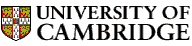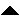Computer LaboratoryComputer Science Syllabus - Computation TheoryComputation TheoryNext: Computer Graphics and Image Up: Lent Term 2006: Part Previous: Compiler Construction   Contents

## Computation Theory

Lecturer: Professor A.M. Pitts

No. of lectures: 12

Prerequisite course: Discrete Mathematics or Mathematics for Computation Theory

This course is a prerequisite for Complexity Theory (Part IB and Diploma), Quantum Computing (Part II and Diploma)

Aims

The aim of this course is to introduce several apparently different formalisations of the informal notion of algorithm; to show that they are equivalent; and to use them to demonstrate that there are uncomputable functions and algorithmically undecidable problems.

Lectures

• Introduction: algorithmically undecidable problems. Decision problems. The informal notion of algorithm, or effective procedure. Examples of algorithmically undecidable problems. [1 lecture]

• Register machines. Definition and examples; graphical notation. Register machine computable functions. Doing arithmetic with register machines. [1 lecture]

• Universal register machine. Natural number encoding of pairs and lists. Coding register machine programs as numbers. Specification and implementation of a universal register machine. [2 lectures]

• Undecidability of the halting problem. Statement and proof. Example of an uncomputable partial function. Decidable sets of numbers; examples of undecidable sets of numbers. [1 lecture]

• Turing machines. Informal description. Definition and examples. Turing computable functions. Equivalence of register machine computability and Turing computability. The Church-Turing Thesis. [2 lectures]

• Primitive recursive functions. Definition and examples. Primitive recursive partial function are computable and total. [1 lecture]

• Partial recursive functions. Definition. Existence of a recursive, but not primitive recursive function. Ackermann's function. A partial function is partial recursive if and only if it is computable. [2 lectures]

• Recursive and recursively enumerable sets. Decidability and recursive sets. Generability and recursive enumeration. Example of a set that is not recursively enumerable. Example of a recursively enumerable set that is not recursive. Alternative characterisations of recursively enumerable sets as the images and the domains of definition of partial recursive functions. [2 lectures]

Objectives

At the end of the course students should

• be familiar with the register machine and Turing machine models of computability

• understand the notion of coding programs as data, and of a universal machine

• be able to use diagonalisation to prove the undecidability of the Halting Problem

• understand the mathematical notion of partial recursive function and its relationship to computability

• be able to develop simple mathematical arguments to show that particular sets are not recursively enumerable

* Hopcroft, J.E., Motwani, R. & Ullman, J.D. (2001). Introduction to automata theory, languages, and computation. Addison-Wesley (2nd ed.).
Cutland, N.J. (1980). Computability. An introduction to recursive function theory. Cambridge University Press.
Davis, M.D., Sigal, R. & Weyuker E.J. (1994). Computability, complexity and languages. Academic Press (2nd ed.).
Sudkamp, T.A. (1995). Languages and machines. Addison-Wesley (2nd ed.).Next: Computer Graphics and Image Up: Lent Term 2006: Part Previous: Compiler Construction   Contents
Christine Northeast
Sun Sep 11 15:46:50 BST 2005© 2005 University of Cambridge Computer Laboratory Please send any comments to pagemaster@cl.cam.ac.uk Page last updated on 11-Sep-2005 at 15:57 by Christine Northeast